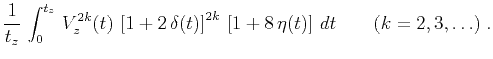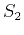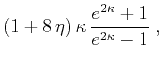Nonhyperbolic reflection moveout of-waves: An overview and comparison of reasonsNext: CURVILINEAR REFLECTOR Up: VERTICAL HETEROGENEITY Previous: Vertically heterogeneous isotropic model

## Vertically heterogeneous VTI model

In a model that includes vertical heterogeneity and anisotropy, both factors influence bending of the rays. The weak anisotropy approximation, however, allows us to neglect the effect of anisotropy on ray trajectories and consider its influence on traveltimes only. This assumption is analogous to the linearization, conventionally done for tomographic inversion. Its application to weak anisotropy has been discussed by Grechka and McMechan (1996). According to the linearization assumption, we can retain isotropic equation (20) describing the ray trajectories and rewrite equation (19) in the form(40)

whereis the anisotropic group velocity, which varies both with the depthand with the ray angleand has the expression (1). Differentiation of the parametric traveltime equations (40) and (20) and linearization with respect to Thomsen's anisotropic parameters shows that the general form of equations (23)-(26) remains valid if we replace the definitions of the root-mean-square velocityand the parametersby(41)(42)

In homogeneous media, expressions (41) and (42) transform series (22) with coefficients (23)-(26) into the form equivalent to series (13). Two important conclusions follow from equations (41) and (42). First, if the mean value of the anisotropic coefficientis less than zero, the presence of anisotropy can reduce the difference between the effective root-mean-square velocity and the effective vertical velocity. In this case, the influence of anisotropy and heterogeneity partially cancel each other, and the moveout curve may behave at small offsets as if the medium were homogeneous and isotropic. This behavior has been noticed by Larner and Cohen (1993). On the other hand, if the anellipticity coefficientis positive and different from zero, it can significantly increase the values of the heterogeneity parametersdefined by equations (29). Then, the nonhyperbolicity of reflection moveouts at large offsets is stronger than that in isotropic media.

To exemplify the general theory, let us consider a simple analytic model with constant anisotropic parameters and the vertical velocity linearly increasing with depth according to the equation(43)

whereis the logarithm of the velocity change. In this case, the analytic expression for the RMS velocityis found from equation (41) to be(44)

while the mean vertical velocity is(45)

whereis evaluated at the reflector depth. Comparing equations (44) and (45), we can see that the squared RMS velocityequals to the squared mean velocityif(46)

For small, the estimate offrom equation (46) is(47)

For example, if the vertical velocity near the reflector is twice that at the surface (i.e.,), having the anisotropic parameteras small asis sufficient to cancel out the influence of heterogeneity on the normal-moveout velocity. The values of parametersand, found from equations (29), (41) and (42), are(48)(49)

Substituting equations (48) and (49) into the estimates (32) and (38) and linearizing them both inand in, we find that the error of anisotropic traveltime approximation (12) in the linear velocity model is(50)

while the error of the shifted-hyperbola approximation (30) is(51)

Comparing equations (50) and (51), we conclude that if the medium is elliptically anisotropic, the shifted hyperbola can be twice as accurate as the anisotropic equation (assuming the optimal choice of parameters). The accuracy of the latter, however, increases when the anellipticity coefficientgrows and becomes higher than that of the shifted hyperbola ifsatisfies the approximate inequality(52)

For instance, if, inequality (52) yields, a quite small value.Nonhyperbolic reflection moveout of-waves: An overview and comparison of reasonsNext: CURVILINEAR REFLECTOR Up: VERTICAL HETEROGENEITY Previous: Vertically heterogeneous isotropic model

2014-01-27